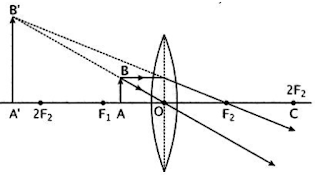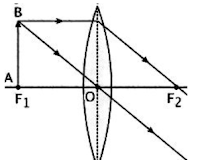#### Extra Questions for Class 10th: Ch 10 Light - Reflection and Refraction (Science) Important Questions Answer Included

Very Short Answer Questions (VSAQs): 1 Mark

Q1. What is magnification of plane mirror?
+1

Q2. When a convex lens will act as magnifying Glass?
When the object is kept on focal length of the convex lens, it is called magnifying glass.

Q3. Name the mirror
(a) Used by dentist
(b) Used for rear view mirror
(a) Concave mirror
(b)  Convex mirror

Q4. The radius of curvature of a convex mirror is 20cm. What is its focal length?
Focal length= 20cm/2 = 10cm

Q5. What is power of Lens of focal length of 20cm?
1/20cm = 1/0.2m=100/20= 5 Diopter.

Short Answer Questions-I (SAQs-I): 2 Marks

Q1. The refractive indices of glass and water with respect to air are 3/2 and 4/3 respectively. If speed of light in glass is 2×108 m/s, find the speed of light in water.

Refractive index of a medium = Speed of light in air/Speed of light in the medium
⇒ 3/2 = Speed of light in air/2×108 m/s
Speed of light in air = 3×108 m/s
Speed of light in water = (3×108 m/s)/(4/3) = 2.25×108 m/s

Q2. What is meant by power of a lens ? Write its SI unit.
The power of a lens is defined as the reciprocal of its focal length (I) expressed in meters. SI unit of power is diopter. One diopter is defined as the power of a lens whose focal length is 1 meter.

Q3. Draw the ray diagram of image formation in following Case? Write size, position and nature of image formed?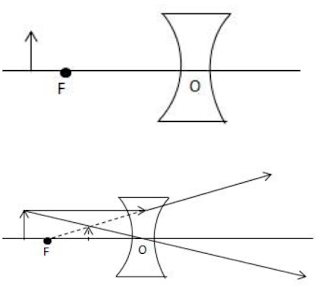Image formed is virtual, erect and demised.

Q4. State two positions in which a concave mirror produces a magnified image of a given object List two difference between them.

 Position Between its pole and focus Between the focus and center of curvature Difference 1 The image is virtual and erect. The image is real and inverted Difference 2 Image is formed behind mirror. The image is formed in front of mirror

Short Answer Questions-I (SAQs-II): 3 Marks

Q1. The image of an object formed by a mirror is real, inverted and is of magnification -1. If the image is at a distance of 40 cm from the mirror, where is the object placed ? Where would the image be if the object is moved 20 cm towards the mirror? State reason and also draw ray diagram for the new position of the object to justify your answer.

The mirror is concave mirror. Since, magnification is -1, it means the size of image is equal to size of object. Also, by the formula of magnification,
M= -v/u = -1
⇒v=u
⇒ (Image distance)=(Object distance)=40cm
This is the case, when object is placed at center of curvature or twice the length of focal length.
So, twice the focal length is 40cm
Focal length= 40cm/2 = 20cm.
When object is placed at focal length, the image is formed at infinity.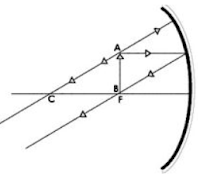Q2. A student wants to project the image of a candle flame on a screen 80 cm in front of a mirror by keeping the candle flame at a distance of 20 m from its pole. (i) Which type of mirror should the student use ? (ii) Find the magnification of the image produced. (iii) Find the distance between the object and its image.

(i) Concave Mirror
(ii) It is given, object distance u=-20m , Image distance v=-80m
Magnification is given as M=-v/u
M=-v/u= -(-80m/-20m) = 4
Distance between object and image  v-u =-80m-(-20m)= 60m

Q3. (i) Name the spherical mirror used as : (a) Shaving mirror (b) Rear view mirror in vehicles (c) Reflector in search-lights. (ii) Write any three differences between a real and a virtual image

Shaving mirror— Concave mirror
Rear view mirror — Convex mirror
Reflector in search-lights — Concave mirror .
Real image can be obtained on screen but virtual image cannot be obtained.
Reflected / Refracted rays actually meet where real image is formed while for virtual they only appear to meet. 'A Real image is always inverted while virtual image is always erect.

Q5. An object is kept at a distance of 18 cm, 20 cm, 22 cm and 30 cm, from a lens of power + 5D. (i) In which case or cases would you get a magnified image ? (ii) Which of the magnified image can we get on a screen ? (b) List two widely used applications of a convex lens.

Focal length = 1/power = 1/5D = 1/5m = 20cm
It is a convex lens of focal length 20cm.
So, magnified image will be formed in all cases, 20cm is focus, 18cm is on focal length, 22cm and 30cm  is between focus and focus and center of curvature. In all cases, magnified image is formed.
(ii) In case of 22cm and 30cm image formed is real and hence can be obtained on screen.

Long Answer Questions (LAQs): 5 Marks

Q1. (a) State the laws of refraction of light. Explain the term absolute refractive index of a medium and write an expression to relate it with the speed of light in vacuum.
(b) The absolute refractive indices of two media 'A' and 'B' are 2.0 and 1.5 respectively. If the speed of light in medium 'B' is 2x108 m/s, calculate the speed of light in : (i) vacuum, (ii) medium 'A'

Laws of Refraction
(i) The incident ray, the refracted ray and the normal to the interface of two transparent media at the point of incidence, all lie in the same plane.
(ii) The ratio of sine of angle of incidence to the sine of angle of refraction is a constant, for the light of a given colour and for the given pair of media. This law is also known as Snell’s law of refraction.
μ=sin⁡i/sin⁡r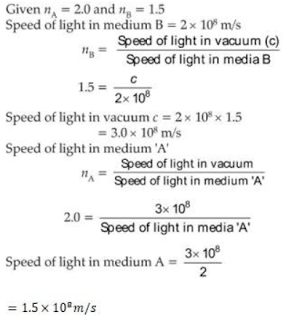Q2. A convex lens can form a magnified erect as well as magnified inverted image of an object placed in front of it". Draw ray diagram to justify this by statement stating the position of the object with respect to the lens in each case. An object of height 4 cm is placed at a distance of 20 cm from a concave lens of focal length 10 cm. Use lens formula to determine the position of the image formed.